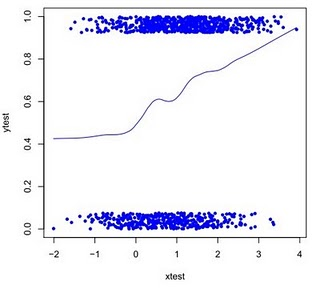Want to share your content on R-bloggers? click here if you have a blog, or here if you don't.

The plot in section 7.3 has some problems. At the very least, the jittered values ought to be between 0 and 1, so the smoothed lines ﬁt better with them. Once again we use the data generated in section 7.2 as an example. For both SAS and R, we use conditioning (section 1.11.2) to make the jitter happen within the 0-1 range.

SAS
In SAS, we use axis statements (section 5.3.8) to clean up the axis tick marks and labels.

`data lp1;set test;jitter = uniform(0) * 0.075;if ytest eq 1 then yplot = ytest - jitter;else if ytest eq 0 then yplot = ytest + jitter;run;axis1 minor = none label = ("xtest");axis2 minor = none label = (angle=270 rotate=90 "ytest");symbol1 i=sm50s v=none c =  blue;symbol2 i=none v=dot h = .2 c = blue;proc gplot data = lp1;plot (ytest yplot) * xtest / overlay haxis=axis1 vaxis=axis2;run;quit;`

And the resulting plot is:R

In R, we add a label to the y axis with the ylab option (section 5.3.8). We also modify the smoother to be a little less responsive to the data (by using a wider window, see section 5.2.6).

`jittery <- jitter(ytest, amount=.0375)correction <- ifelse(ytest==0, .0375, -.0375)jittery <- jittery + correctionplot(xtest, ytest, type="n")points(xtest, jittery, pch = 20, col = "blue", ylab = "ytest")lines(lowess(xtest, ytest, f = .4), col = "blue") `

And the resulting plot is:As with the uglier version shown in example 7.3, the differences between the two plots results from there being different randomly-generated data sets and because we use two different smoothers.

In the next example, we’ll show how to make a SAS Macro or an R function to replicate this plot easily.